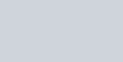Reduce the equation of the plane
Question:

Reduce the equation of the plane $4 x-3 y+2 z=12$ to the intercept form, and hence find the intercepts made by the plane with the coordinate axes.

Solution:$\therefore \frac{x}{3}+\frac{y}{-4}+\frac{z}{6}=1$